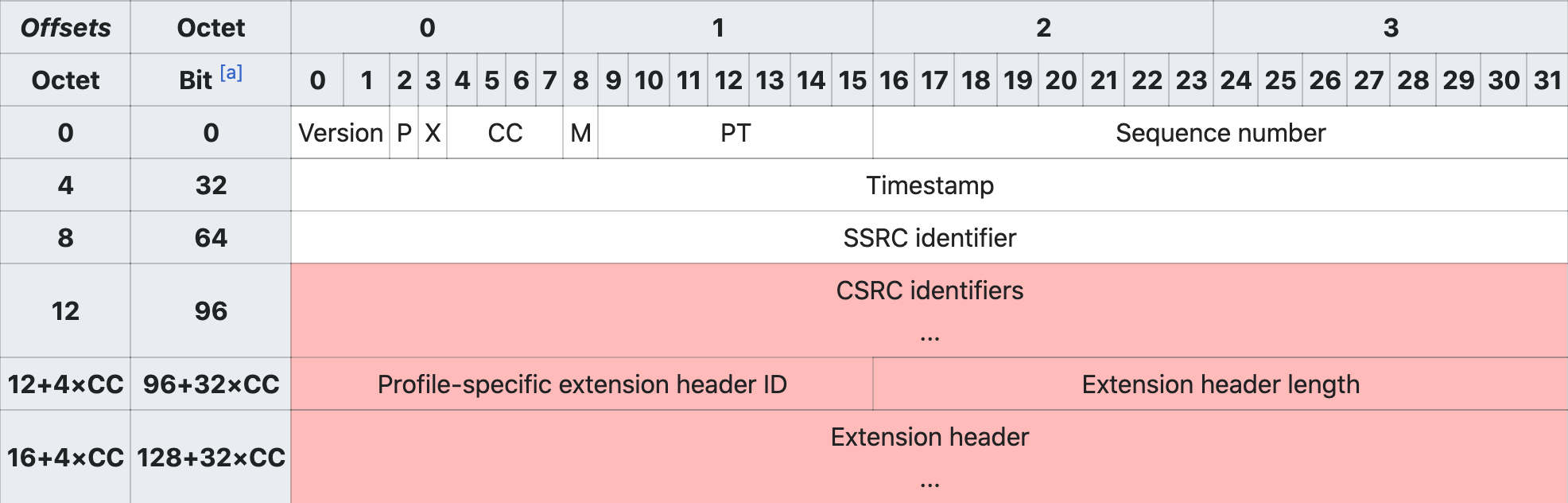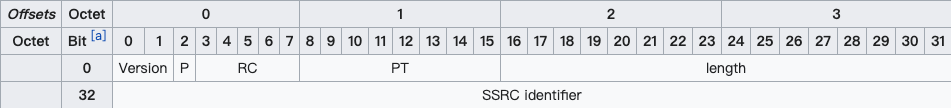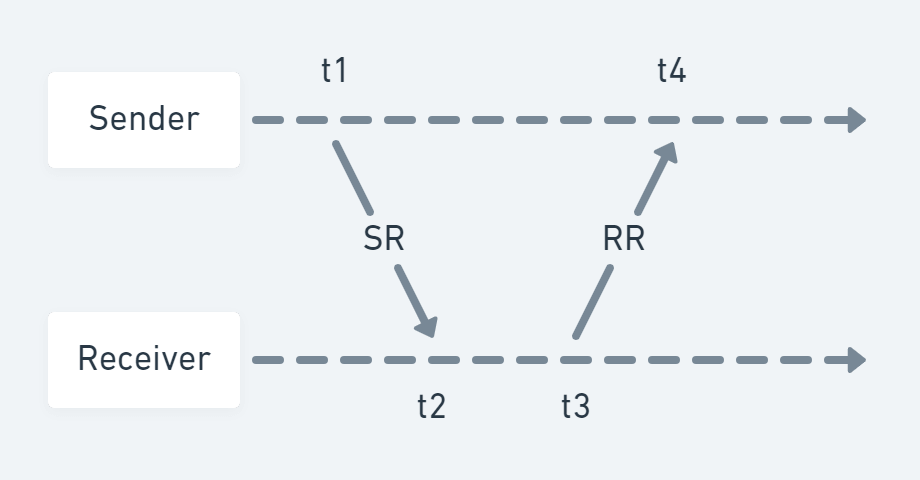RTP 协议介绍

Data Transfer & Control 📝

WebRTC 使用 RTP 协议传输音视频，也依赖于 RTP 协议提供的信息处理丢包。本文尝试结合 RFC 3350 对 RTP 协议做一个较为简洁的介绍，方便大家查阅。

RTP（Real-time Transport Protocol，实时传输协议）是一种运行在 OSI 应用层的协议，通常基于 UDP 协议，但也支持 TCP 协议。它提供了端到端的实时传输数据的功能，但不包含资源预留存 1（resource reservation）、不保证实时传输质量，这些功能都需要 WebRTC 自己实现。

RTP 协议分为两种子协议，分别是 RTP Data Transfer Protocol 和 RTP Control Protocol。前者顾名思义，是用来传输实时数据的；后者则是我们常说的 RTCP 协议，可以提供实时传输过程中的统计信息（如网络延迟、丢包率等），WebRTC 正是根据这些信息处理丢包。

RTP Data Transfer Protocol• Version 表示 RTP 协议的版本，目前版本为 2。
• X (Extension) 表示是否有头部扩展，头部扩展可以用于存储信息，比如视频旋转角度。
• CC (CSRC count) 表示红色部分的 CSRC（参见下文）数量，显然最多只能有 15 个 CSRC。
• M (Marker) 表示当前数据是否与应用程序有某种特殊的相关性。比如传输的是一些私有数据，或者数据中的某些标志位具有特殊的作用。
• Sequence number 是递增的序列号，用于标记每一个被发送的 RT(D)P 包。接收方可以根据序列号按顺序重新组包，以及识别是否有丢包。序列号的初始值应当是随机的（不可预测的），从而增加明文攻击的难度。
• Timestamp 即时间戳，接收方根据其来回放音视频。时间戳的间隔由传输的数据类型（或具体的应用场景）确定，比如音频通常以 125µs（8kHz）的时钟频率进行采样，而视频则以 90kHz 的时钟频率采样。这里时间戳的初始值也是随机选取的，是一种相对时间戳。
• SSRC (Synchronization source) 即同步源标识符。相同 RTP 会话中的 SSRC 是唯一的，且生成的 SSRC 也需要保持随机。尽管多个源选中同一个标识符的概率很低，但具体实现时仍然需要这种情况发生，即避免碰撞。
• CSRC (Contributing source) 在 MCU 混流时使用，表示混流出的新的音视频流的 SSRC 是由哪些源 SSRC 贡献的。根据上述 CC 得知，我们最多可以同时混 15 路音视频流。

获取视频旋转角度RtpPacket.hpp
// class RtpPacket
bool ReadVideoOrientation(bool& camera, bool& flip, uint16_t& rotation) const
{
// 从 extention header 中解析出视频旋转方向的 extenValue
uint8_t extenLen;
uint8_t* extenValue = GetExtension(this->videoOrientationExtensionId, extenLen);

if (!extenValue || extenLen != 1u)
return false;

// 从 extenValue 对应的 bit 上获取对应的值
uint8_t cvoByte       = Utils::Byte::Get1Byte(extenValue, 0);
uint8_t cameraValue   = ((cvoByte & 0b00001000) >> 3);
uint8_t flipValue     = ((cvoByte & 0b00000100) >> 2);
uint8_t rotationValue = (cvoByte & 0b00000011);

camera = cameraValue ? true : false;
flip   = flipValue ? true : false;

// 视频逆时针旋转的角度，即需要将视频顺时针旋转多少度才是屏幕自然方向
switch (rotationValue)
{
case 3:
rotation = 270;
break;
case 2:
rotation = 180;
break;
case 1:
rotation = 90;
break;
default:
rotation = 0;
}

return true;
}

RTP Control Protocol (RTCP)

RTCP 协议提供实时传输过程中的统计信息，如网络延迟、丢包率等。在传统的实时通讯过程中，RT(D)P 协议占用偶数位的端口，而 RTCP 协议占用随后的奇数位端口。不过如果接收方的 SDP 中包含 rtcp-mux 字段 6，即表明接收方支持 RT(D)P 协议和 RTCP 协议共用同一个端口，即多路复用。在 Chrome 57 版本已经强制开启了 rtcp-mux• RC (Reception report count) 表示当前 RTCP 包有几个 block，显然最多只能有 32 个。
• PT (Packet type) 表示 RTCP 包的类型，比如 SR=200、RR=201（SR、RR 参见下文）。
• Length 等于整个 RTCP 包的长度减一（使得 Length = 0 是合法的），其值包含 header 的长度和所有 padding 占用的空间长度。值的单位是以 32 位字长（32-bit words）描述的。

SR & RR

PT=SR=200 时，表示这个 RTCP 包记录的是发送方的质量数据（Sender Report）；PT=RR=201 时，表示这个 RTCP 包记录的是接收方的质量数据（Receiver Report）。SR 和 RR 的结构几乎一致，除了 RR 没有 sender info。先来看 SR 的结构：

0                   1                   2                   3
0 1 2 3 4 5 6 7 8 9 0 1 2 3 4 5 6 7 8 9 0 1 2 3 4 5 6 7 8 9 0 1
+-+-+-+-+-+-+-+-+-+-+-+-+-+-+-+-+-+-+-+-+-+-+-+-+-+-+-+-+-+-+-+-+
header |V=2|P|    RC   |   PT=SR=200   |             length            |
+-+-+-+-+-+-+-+-+-+-+-+-+-+-+-+-+-+-+-+-+-+-+-+-+-+-+-+-+-+-+-+-+
|                         SSRC of sender                        |
+=+=+=+=+=+=+=+=+=+=+=+=+=+=+=+=+=+=+=+=+=+=+=+=+=+=+=+=+=+=+=+=+
sender |              NTP timestamp, most significant word             |
info   +-+-+-+-+-+-+-+-+-+-+-+-+-+-+-+-+-+-+-+-+-+-+-+-+-+-+-+-+-+-+-+-+
|             NTP timestamp, least significant word             |
+-+-+-+-+-+-+-+-+-+-+-+-+-+-+-+-+-+-+-+-+-+-+-+-+-+-+-+-+-+-+-+-+
|                         RTP timestamp                         |
+-+-+-+-+-+-+-+-+-+-+-+-+-+-+-+-+-+-+-+-+-+-+-+-+-+-+-+-+-+-+-+-+
|                     sender's packet count                     |
+-+-+-+-+-+-+-+-+-+-+-+-+-+-+-+-+-+-+-+-+-+-+-+-+-+-+-+-+-+-+-+-+
|                      sender's octet count                     |
+=+=+=+=+=+=+=+=+=+=+=+=+=+=+=+=+=+=+=+=+=+=+=+=+=+=+=+=+=+=+=+=+
report |                 SSRC_1 (SSRC of first source)                 |
block  +-+-+-+-+-+-+-+-+-+-+-+-+-+-+-+-+-+-+-+-+-+-+-+-+-+-+-+-+-+-+-+-+
1    | fraction lost |       cumulative number of packets lost       |
+-+-+-+-+-+-+-+-+-+-+-+-+-+-+-+-+-+-+-+-+-+-+-+-+-+-+-+-+-+-+-+-+
|           extended highest sequence number received           |
+-+-+-+-+-+-+-+-+-+-+-+-+-+-+-+-+-+-+-+-+-+-+-+-+-+-+-+-+-+-+-+-+
|                      interarrival jitter                      |
+-+-+-+-+-+-+-+-+-+-+-+-+-+-+-+-+-+-+-+-+-+-+-+-+-+-+-+-+-+-+-+-+
|                         last SR (LSR)                         |
+-+-+-+-+-+-+-+-+-+-+-+-+-+-+-+-+-+-+-+-+-+-+-+-+-+-+-+-+-+-+-+-+
|                   delay since last SR (DLSR)                  |
+=+=+=+=+=+=+=+=+=+=+=+=+=+=+=+=+=+=+=+=+=+=+=+=+=+=+=+=+=+=+=+=+
report |                 SSRC_2 (SSRC of second source)                |
block  +-+-+-+-+-+-+-+-+-+-+-+-+-+-+-+-+-+-+-+-+-+-+-+-+-+-+-+-+-+-+-+-+
2    :                               ...                             :
+=+=+=+=+=+=+=+=+=+=+=+=+=+=+=+=+=+=+=+=+=+=+=+=+=+=+=+=+=+=+=+=+
|                  profile-specific extensions                  |
+-+-+-+-+-+-+-+-+-+-+-+-+-+-+-+-+-+-+-+-+-+-+-+-+-+-+-+-+-+-+-+-+

• NTP timestamp 即 NTP 时间戳。完整的 NTP 时间戳为 64 位无符号定点（fixed-point）数，可以和 RR 中的时间戳计算出对应接收方的 Round-Trip Time（RTT）。
• RTP timestamp 对应上述 NTP 时间戳的同一时间，但是与 RT(D)P 时间戳的单位和偏移量保持一致。这种对应关系可以用于媒体源的帧间或帧内同步（如果它们的 NTP 时间戳也是同步的话），也可以被媒体无关（media-independent）的接收方用于估算 RTP 时钟频率。
• sender’s packet count 即截止到当前 SR 生成时，发送方一共传输了多少个 RT(D)P 包。如果发送方的 SSRC 改变了，则该计数值将会被重置。

SR 和 RR 具有相同的 report block 结构，其中各个字段的含义分别如下：

1. fraction lost 表示最近一次 SR 或 RR 发送后，对应 SSRC 的发送方的 RT(D)P 丢包率，为 8 位定点数，换算为百分比的公式为 percentage = fractionLost * 100 / 256 。值等于发送方实际丢包数（参见下文）除以接收方期望收到的包数量（参见下文）。
2. packets lost 表示截止到当前实时通话时，对应 SSRC 的发送方的 RT(D)P 累计丢包数。值等于接收方期望收到的包数量减去实际收到的包数量，即上述「发送方实际丢包数」。
3. extended highest sequence number received 低 16 位为接收方从对应 SSRC 的发送方收到的最大的 RT(D)P sequence number，高 16 位为对应的 sequence number 循环次数。而对应的计算值便是上述「接收方期望收到的包数量」。
4. interarrival jitter 为接收方收到 RT(D)P 包的间隔时间的统计方差，为无符号整数时间值。
5. LSR 表示接收方收到对应 SSRC 的发送方的最近一次 SR 中的 NTP 时间戳，采用的是更加紧凑的 32 位表示方法。如果没有 SR 被收到过，则这个值为零。
6. DLSR 表示接收方收到对应 SSRC 的发送方的最近一次 SR 的时间，和接收方发送 RR 的时间差，单位为 1/65536 秒。如果没有 SR 被收到过，则这个值为零。

Round-Trip Time1. 参见维基百科 资源预留协议

2. 引用自维基百科 Real-time Transport Protocol

3. 只有刚连接时的 RT(D)P 包和视频发生旋转时的 RT(D)P 包才会携带旋转角度信息

4. 完整代码可以参见 RtpPacket.hpp#L432

5. 引用自维基百科 RTP Control Protocol